×#### Thank you for registering.

One of our academic counsellors will contact you within 1 working day.

Click to Chat

1800-1023-196

+91-120-4616500

CART 0

• 0

MY CART (5)

Use Coupon: CART20 and get 20% off on all online Study Material

ITEM
DETAILS
MRP
DISCOUNT
FINAL PRICE
Total Price: Rs.

There are no items in this cart.
Continue Shopping• Complete Physics Course - Class 11
• OFFERED PRICE: Rs. 2,968
• View Details

Diffraction

Table of Content

Diffraction Pattern from a Single Slit

Fresnel and Fraunhofer Diffraction

Diffraction Grating

Theory

Related ResourcesSound is propagated in the form of waves. Sound produced in an adjoining room reaches us after bending round the edges of the walls. Similarly, waves on the surface of water also bend round the edges of an obstacle and spread into the region behind it. This bending of waves around the edges of an obstacle is called diffraction. Diffraction is a characteristic property of waves. The waves are diffracted, only when the size of the obstacle is comparable to the wavelength of the wave.

Fresnel showed that the amount of bending produced at an obstacle depends upon the wavelength of the incident wave. Since the sound waves have a greater wavelength, the diffraction effects are pronounced. As the wavelength of light is very small, compared to that of sound wave and even tiny obstacles have large size, compared to the wavelength of light waves, diffraction effects of light are very small.

In practice, diffraction of light can be observed by looking at a source of white light through a fine piece of cloth. A series of coloured images are observed.

Diffraction Pattern from a Single Slit

The sketch shows the view from above a single slit. Let's assume that the slit is constant width and very tall compared with that width, so that we can consider the system as two-dimensional. With light at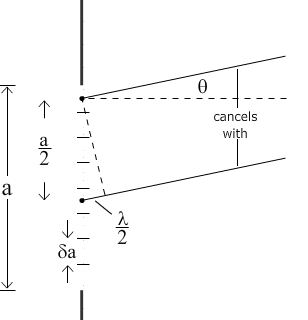normal incidence, the pattern is symmetrical about the axis of the slit. On a distant screen, the light arriving on the axis from all points in the slit has travelled an equal distance from the slit, so the centre of the pattern is a maximum. The next question is what determines its width.

Notice the broad central maximum, and the equally spaced, successively weaker maxima on either side.

This animated sketch shows the angle of the first order minima: the first minimum on either side of the central maximum. We call the slit width a, and we imagine it divided into two equal halves. Using the Huygen’s construction, we consider a point at the very top of the slit, and another point a distance a/2 below it, i.e. a point at the very top of the lower half of the slit. Consider parallel rays from both points, at angle θto the axis of symmetry. (Why parallel? Because the screen is distant. Typically in diffraction experiments, the slit is ~ 10 µm wide, while the distance to the screen might be ~ 1 m.)

The ray from the distance a/2 below has to travel an extra distance (a sin θ/2). If this distance is half a wavelength, i.e. if a sin θ  =  λ, then they are π/2 out of phase and they interfere destructively. Now, for every point in the top half of the slit, there is one in the bottom half a distance a/2 below and, at the angle that satisfies a sin θ  =  λ, they all interfere destructively.

So the first minimum has sin θ  =  λ/a. On the other side of the axis of symmetry, sin θ  =  –λ/a is also a minimum. These two minima limit the broad central maximum.

Fresnel and Fraunhofer Diffraction

Diffraction  phenomenon  can  be  classified  under  two  groups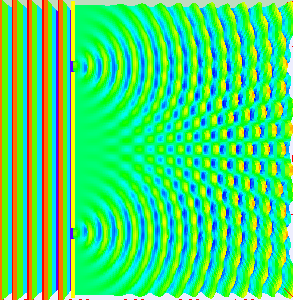(i) Fresnel diffraction and (ii) Fraunhofer diffraction. In the Fresnel diffraction, the source and the screen are at finite distances from the obstacle producing diffraction. In such a case the wave front undergoing diffraction is either spherical or cylindrical. In the Fraunhofer diffraction, the source and the screen are at infinite distances from the obstacle producing diffraction. Hence in this case the wave front undergoing diffraction is plane. The diffracted rays which are parallel to one another are brought to focus with the help of a convex lens. Fraunhofer pattern is easier to observe practically by a spectrometer.

Diffraction Grating

An arrangement consisting of a large number of equidistant parallel narrow slits of equal width separated by equal opaque portions is known as a diffraction grating.

The plane transmission grating is a plane sheet of transparent material on which opaque rulings are made with a fine diamond pointer. The modern commercial form of grating contains about 6000 lines per centimeter.

The rulings act as obstacles having a definite width ‘b’ and the transparent space between the rulings act as slit of width ‘a’. The combined width of a ruling and a slit is called grating element (e). Points on successive slits separated by a distance equal to the grating element are called corresponding points.

Theory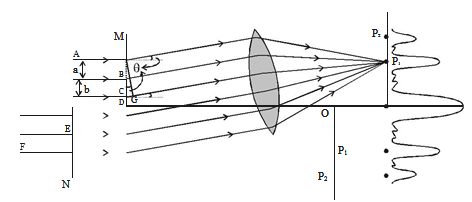MN represents the section of a plane transmission grating. AB, CD, EF … are the successive slits of equal width a and BC, DE … be the rulings of equal width b as shown in figure. Let e = a + b.

Let a plane wave front of monochromatic light of wave length λ be incident normally on the grating. According to Huygen’s principle, the points in the slit AB, CD … etc act as a source of secondary wavelets which spread in all directions on the other side of the grating.

Let us consider the secondary diffracted wavelets, which makes an angle θ with the normal to the grating.

The path difference between the wavelets from one pair of corresponding points A and C is CG = (a + b) sin θ. It will be seen that the path difference between waves from any pair of corresponding points is also (a + b) sin θ.

The point P1 will be bright, when

(a + b) sin θ = m λ where m = 0, 1, 2, 3

In the un diffracted position θ = 0 and hence sin θ = 0.

(a + b) sin θ = 0, satisfies the condition for brightness for m = 0. Hence the wavelets proceeding in the direction of the incident rays will produce maximum intensity at the centre O of the screen. This is called zero order maximum or central maximum.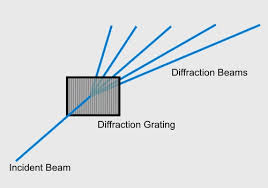If (a + b) sin θ1 = λ, the diffracted wavelets inclined at an angle θ1 to the incident direction, reinforce and the first order maximum is obtained.

Similarly, for second order maximum, (a + b) sin θ2 = 2λ

On either side of central maxima different orders of secondary maxima are formed at the point P1, P2.

In general, (a + b) sin θ = m λ is the condition for maximum intensity, where m is an integer, the order of the maximum intensity.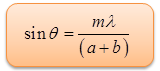Or, sin θ = Nmλ

Here N = 1/a+b, gives the number of grating element or number of lines per unit width of the grating.

When white light is used, the diffraction pattern consists of a white central maximum and on both sides continuous coloured images are formed.

In the un diffracted position, θ = 0 and hence sin θ = 0. Therefore sin θ = Nmλ is satisfied for m= 0 for all values of λ. Hence, at O all the wavelengths reinforce each other producing maximum intensity for all wave lengths. Hence an undispersed white image is obtained.

As θ increases, (a + b) sin θ first passes through λ/2 values for all colours from violet to red and hence darkness results. As θ further increases, (a + b) sin θ passes through λ values of all colours resulting in the formation of bright images producing a spectrum from violet to red. These spectra are formed on either side of white, the central maximum.

Watch this Video for more referenceThis phenomenon can also occur when light is "bent" around particles that are on the same order of magnitude as the wavelength of the light.

The amount of diffraction depends on the wavelength of light, with shorter wavelengths being diffracted at a greater angle than longer ones (in effect, blue and violet light are diffracted at a higher angle than is red light)

Diffraction refers to various phenomena that occur when a wave encounters an obstacle. In classical physics, the diffraction phenomenon is described as the apparent bending of waves around small obstacles and the spreading out of waves past small openings

Diffraction is the breaking up of an electromagnetic wave as it passes a geometric structure (e.g., a slit), followed by reconstruction of the wave by interference.

The light waves are diffracted only when the size of the obstacle is comparable to the wavelength of the light. The size of obstacle/slit shouldn’t be large in size.

In Fresnel class of diffraction, the source and/or screen are at a finite distance from the aperture.

In Fraunhoffer class of diffraction, the source and screen are at infinite distance from the diffracting aperture. Fraunhoffer is a special case of Fresnel diffraction

In double slit experiment d/D = 10-4 (d = distance between slits, D = distance of screen from the slits). At a point P on the screen resulting intensity is equal to the intensity due to individual slit I0. Then the distance of point P from the central maximum is (λ = 6000 Å)

(a) 2 mm        (b) 1 mm

(c) 0.5 mm      (d) 4 mm

Solution:

We know that, I = 4I0 cos2 (φ/2)

I0 = 4I0 cos2 (φ/2)

So, cos (φ/2) = ½

Or, φ/2 = π/3

Or, φ = 2π/3 = (2π/λ) . ?x

Or, 1/3 = (1/λ) y (d/D)       (?x = yd/D)

Thus, y = [λ]/[3(d/D)] = 610-7/ 310-7

= 210-3 m = 2 mm

Therefore from the above observation, we conclude that, option (a) is correct.Question 1

A single slit diffraction pattern is obtained on a screen using yellow light. If the yellow light is replaced by blue light without making any other changes in the experimental set up, what will happen to the diffraction bands?

(a) Bands will disappear

(b) Bands will become broader and farther apart

(c) Bands will become broader and crowded together

(d) Bands will become narrower and crowded together

Question 2

Phase difference between two coherent sources should be:

(c) π rad     (d) All the above are correct

Question 3

Two coherent monochromatic light beams of intensities I and 4I are superposed. The maximum and minimum possible intensities in the resulting beams are

(a) 5I and I        (b) 9I and I

(c) 5I and 3I      (d) 9I and 3I

Question 4

Monochromatic light from a narrow slit illuminates two narrow parallel slits producing an interference pattern on a screen. The separation between the two slits is now doubled and the distance between the screen and the slits is reduced to half. The fringe width

(a) is doubled                  (b) becomes four times

(c) becomes one fourth     (d) remains the same

Question 5

One important similarity between sound and light waves is that both

(a) can pass through even in the absence of any medium

(b) are transverse waves

(c) travel at the same speed in air

(d) can show interference effectsQ.1
Q.2
Q.3
Q.4
Q.5

d

b

b

c

d

Related Resources

You might like to polarisation.

For getting an idea of the type of questions asked, refer the  Previous Year Question Papers.### Course Features

• 101 Video Lectures
• Revision Notes
• Previous Year Papers
• Mind Map
• Study Planner
• NCERT Solutions
• Discussion Forum
• Test paper with Video Solution